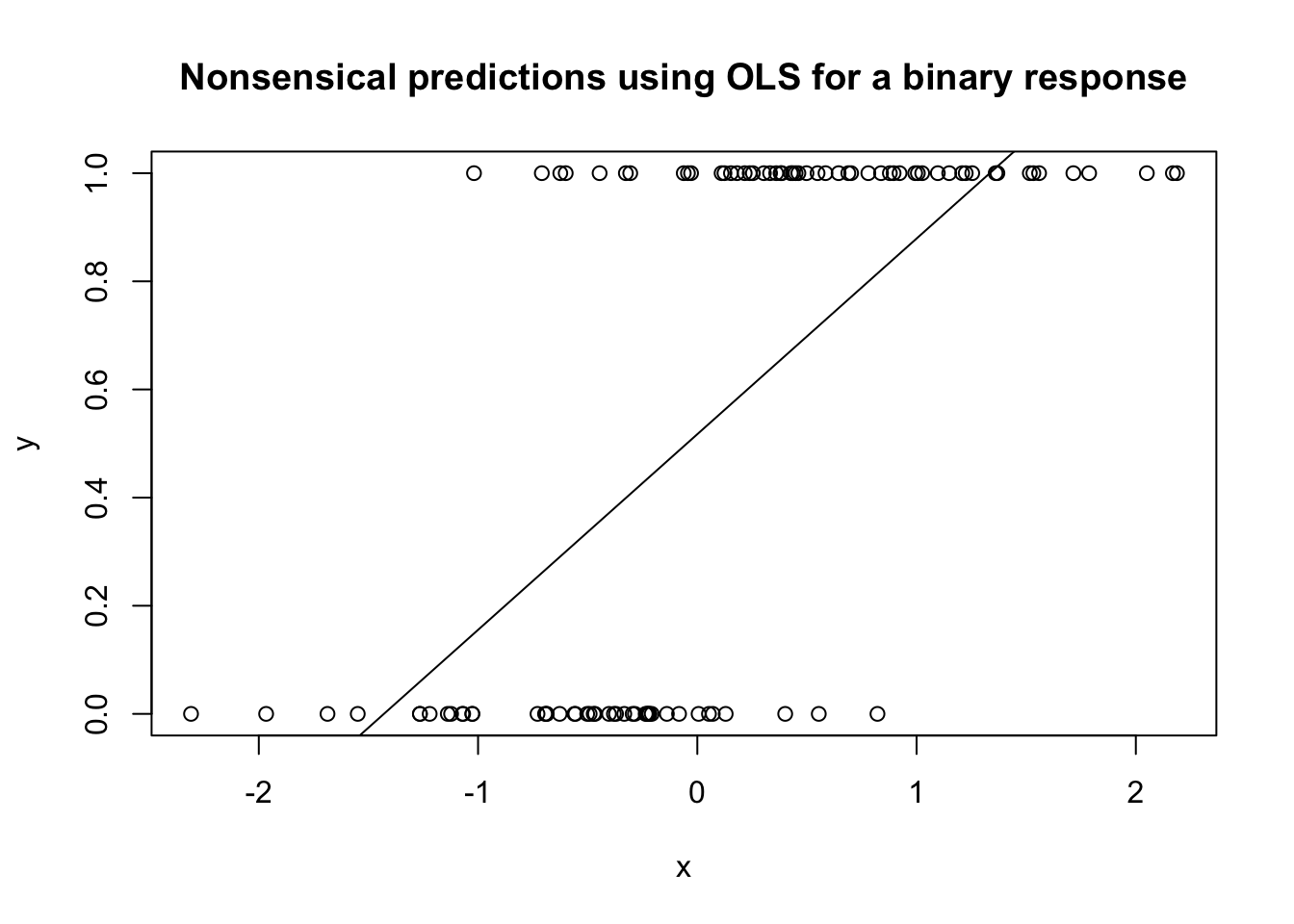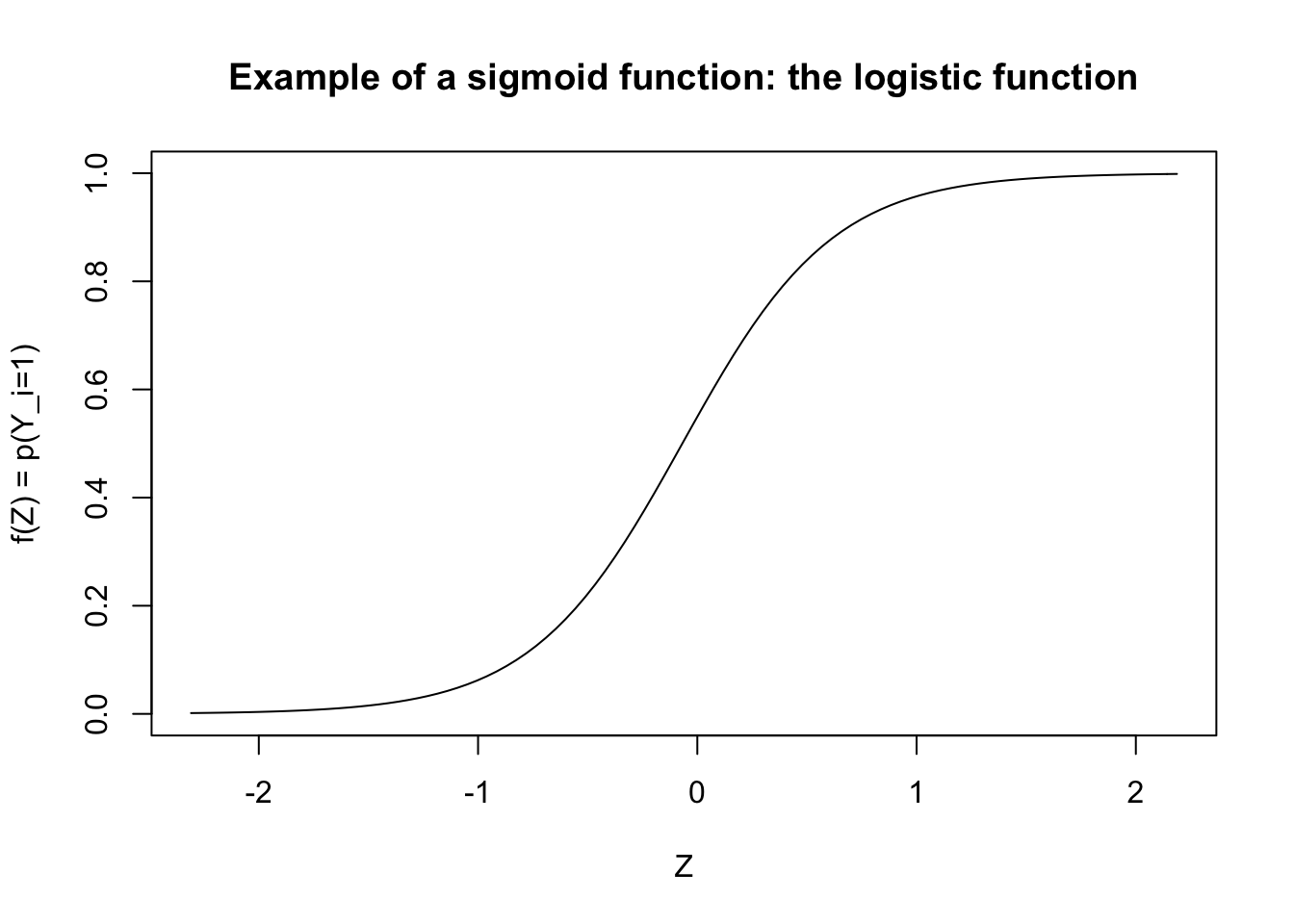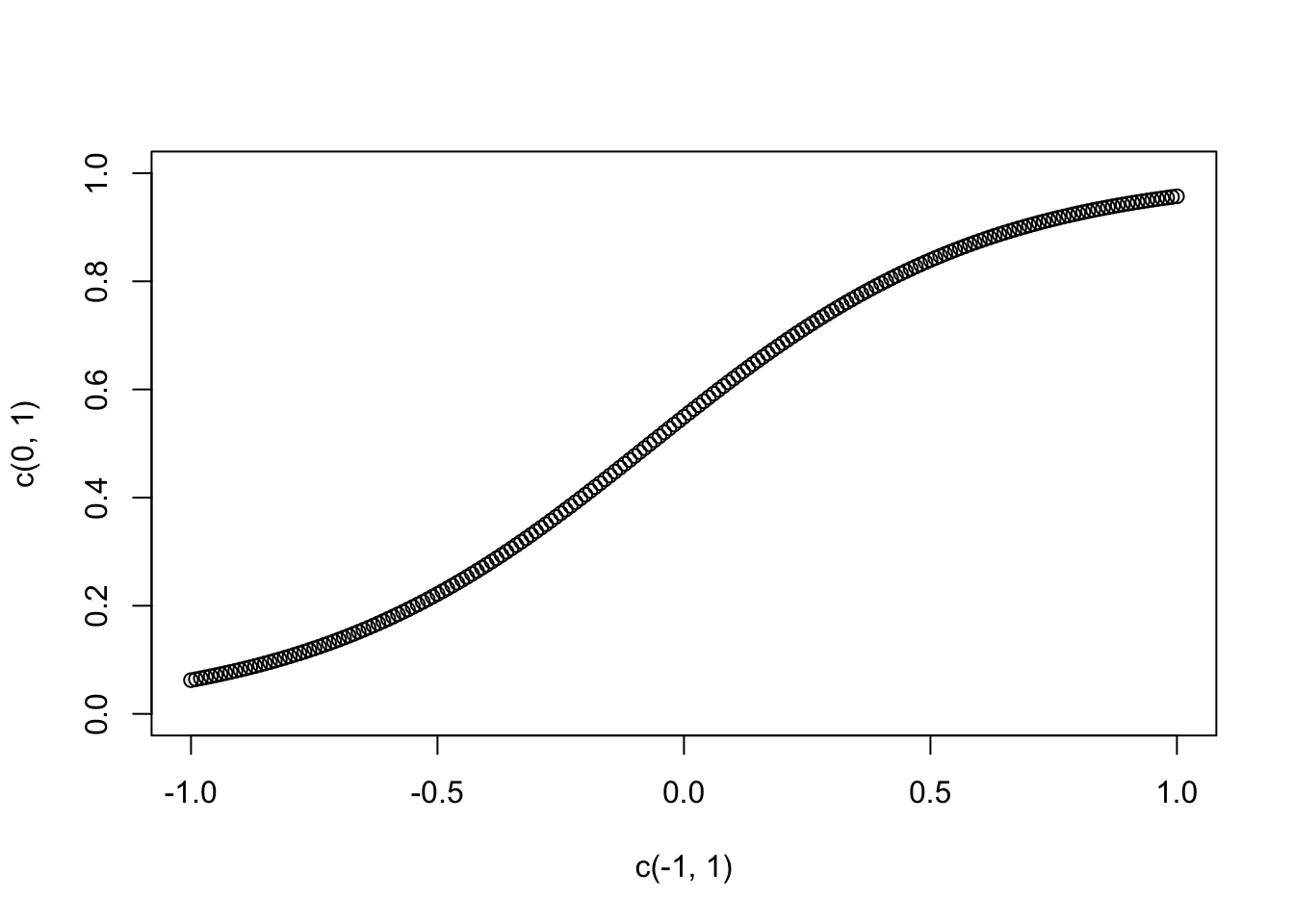# 1 General review of Logits

One of our key goal in this class will be to classify observations into groups–typically event/no event. I.e., we are interested in converting existing information (variables) into a binary classification. There are a number of ways of doing this, but an important one is the logit.

## 1.1 Why logits?

Suppose $$y_i$$ denotes whether a country goes to war or not. I.e., $$y_i=0$$ if it does not enter the war, and $$y_i=1$$ if it does. Suppose that we are interested in the probability that a country goes to war, i.e., $$P(y_i=1)$$. We think the level of trade dependence of that country might explain the decision to go to war. So we estimate the following model: $y_i = \beta_0 + \beta_1 trade_i + \varepsilon_i$

set.seed(123)
n <- 100
x <- rnorm(n, mean=0)
y <- round(1 / (1 + exp(-(x + rnorm(n, sd = 0.5)))))

#--- First use the linear model
lm1 <- lm(y ~ x)
plot(x, y, lty = 2, main='Nonsensical predictions using OLS for a binary response')
abline(lm1)## 1.2 The logit to the rescue

What if, instead, we chose a sigmoid function?

glm.logit <- glm(y ~ x, family = binomial(link = 'logit'))
plot(x, y, type='n', xlab='Z', ylab='f(Z) = p(Y_i=1)', main='Example of a sigmoid function: the logistic function')
curve(predict(glm.logit ,data.frame(x=x),type="resp"), add=TRUE)I.e., let $$Z$$ be a linear function of the $$Xs$$. For example, $Z = \beta_0 + \beta_1, X$ and then we can define $$p(Y_i=1)$$ as a sigmoid function of $$Z$$.

There are two popular versions of this curve: the logistic function, used for the logit, and the cumulative normal distribution, used in probit estimation. Neither has any particular advantage, and it does not really matter which one you use.

In logit estimation the probability of the occurrence of the event is determined by the function \begin{align} p(Y_i=1 | X_i) = f(Z)= \frac{1}{1+e^{-Z}} = \frac{1}{1+e^{-(X\beta)}}\label{eqn:logit} \end{align}

Note that this is simply choosing a different link from $$X$$ to $$p$$. In the OLS case, the link was linear, i.e., $$p(Y_i=1 | X_i) = f(Z)= Z =X\beta$$. With the logit (and similarly with the probit), the link is a sigmoid function, such that as Z increases (to infinity), $$e^{-Z}$$ tends to 0, and hence $$p(Y_i=1 | X_i)$$ tends to 1. Similarly, as Z decreases (to minus infinity), $$e^{-Z}$$ tends to infinity, and hence $$p(Y_i=1 | X_i)$$ tends to 0. As a result, our predictions are bounded by 0 and 1.

## 1.3 Interpretation of logistic coefficients

VERY IMPORTANT: you can no longer interpret $$\beta_k$$ as the marginal effect of variable $$x_k$$ on $$y$$!

To interpret the effect of a change of $$x_k$$ by one unit, we need to calculate the derivative of $$p(Y_i=1 |x_i)$$ with respect to $$x_k$$: $\frac{\partial p(Y_i=1|x_i)}{\partial x_{ik}} = \frac{e^{x_i \beta}}{(1+e^{x_i \beta})^2}\beta_k$ Note that the marginal effect of $$x_{ik}$$ depends on all the $$x_i$$s. I.e., you can no longer say an increase in x leads to an increase in $$y$$ by such amount. Rather, the effect depends on the values of the other covariates. Look back at the figure of the logit curve, and note that the effect is much strong around $$x=0$$ than for $$x=-10$$ or $$x=10$$.

Another way of seeing the same thing is to look at what happens to $$p$$ as X increases by one. Assuming all the betas are 1 for simplicity: $p(Y=1|x_1=0; x_2=0) = \frac{1}{1+e^{-(\beta_1x_1 + \beta_2x_2)}}=0.5$ $p(Y=1|x_1=1; x_2=0) = \frac{1}{1+e^{-(\beta_1x_1 + \beta_2x_2)}}=0.73$

But note also that the effect is different if $$x_2$$ is different: $p(Y=1|x_1=0; x_2=1) = \frac{1}{1+e^{-(\beta_1x_1 + \beta_2x_2)}}=0.73$ $p(Y=1|x_1=1; x_2=1) = \frac{1}{1+e^{-(\beta_1x_1 + \beta_2x_2)}}=0.88$

Suppose for example that you estimate your logistic regression as: $-10+ .16x_1 + 0.02x_2$ Then the effect on the odds of a unit increase in $$x_1$$ is $$e^{0.16}=1.18$$, meaning that the odds increase by 18% regardless of the value of $$x$$

We can plot the effect of $$X$$ on $$Y$$:

plot(c(-1,1), c(0,1), type='n')
for(i in seq(-1,1, 0.01)){
points(i, predict(glm.logit, newdata=data.frame(x = i), type='response'), xlab='x', ylab=' predicted probabilities.')
}The best way to present your results is to plot or report predicted probabilities. However, you always need to be aware that you need to hold all other variables at a given level. The level you choose will affect the effect!! Typically what is done is to hold variables at the median, vary x, and report predicted probability. Or hold variables at the median and set x to its 25 percentile, 50 percentiles, and 75 percentile.

# 2 Replication of Goldsmith et al. 2013

Note: from Goldstein: Our approach is to first estimate the likelihood of instability for a given year, then incorporate this estimate as a predictor variable in a second model estimating the likelihood of genocide onset. We use probit regression models, a familiar, appropriate choice for modeling binary outcomes, at each stage.’’

## 2.1 Preparatory steps

# Change directory
library(stargazer)
##
## Please cite as:
##  Hlavac, Marek (2018). stargazer: Well-Formatted Regression and Summary Statistics Tables.
##  R package version 5.2.2. https://CRAN.R-project.org/package=stargazer
library(foreign) # library to load Stata datasets

gen <- read.dta('ReplicastionMaterialsGBSS_JPR/GBSSgenocideforecastingdatasetJPR2013.dta')

## 2.2 Run the first probit: DV = instability

glm1 <- glm(finstability ~ instability +  RulingEliteEthnicity+ polity2sq_inv +regchange1to3 +  lIMR +   lAssassinDum  +lntotalpop +  ef+ efNELDAperiod+ NELDAperiod+   nac + mena+   csasia+ SLDdum+  stabyrs+ stabyrs2,
data = gen[gen$year > 1973 & gen$year < 1988,],
na.action=na.exclude)
stargazer(glm1, type='html')
 Dependent variable: finstability instability 3.218*** (0.236) RulingEliteEthnicity -0.075 (0.162) polity2sq_inv 0.007** (0.003) regchange1to3 0.076*** (0.017) lIMR 0.003 (0.002) lAssassinDum 0.488** (0.204) lntotalpop 0.106 (0.065) ef 1.076* (0.595) efNELDAperiod -0.156 (0.682) NELDAperiod 0.254 (0.416) nac -0.063 (0.066) mena 0.700*** (0.226) csasia 0.561* (0.307) SLDdum 0.475*** (0.159) stabyrs -0.065** (0.029) stabyrs2 0.002** (0.001) Constant -4.917*** (1.210) Observations 1,638 Log Likelihood -158.387 Akaike Inf. Crit. 350.775 Note: p<0.1; p<0.05; p<0.01
# Predict in-sample
gen$pr_instab[gen$year > 1973 & gen$year < 1988] <- predict(glm1, type='response') # Create a binary version in order to create a confusion matrix (not necessary, but for illustration purposes) gen$pr_instab_binary <- 0
gen$pr_instab_binary[gen$pr_instab >= 0.5] <- 1

# Create the confusion matrix (manually here, but there are packages that'll do this automatically)
print(length(gen$pr_instab_binary[gen$finstability==0 & gen$pr_instab_binary==0]))  5068 print(length(gen$pr_instab_binary[gen$finstability==0 & gen$pr_instab_binary==1]))

 17

print(length(gen$pr_instab_binary[gen$finstability==1 & gen$pr_instab_binary==0]))  756 print(length(gen$pr_instab_binary[gen$finstability==1 & gen$pr_instab_binary==1]))

 291

# generate an ROC curve
library(ROCR) # Load the package that automates the create of the ROC curve
## Loading required package: gplots
##
## Attaching package: 'gplots'
## The following object is masked from 'package:stats':
##
##     lowess
pred <- prediction( gen$pr_instab, gen$finstability)
perf <- performance(pred,"tpr","fpr")
plot(perf)